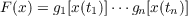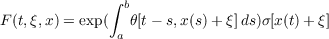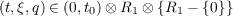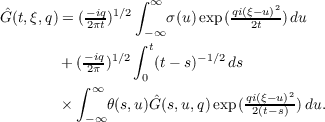#### Vol. 26, No. 1, 1968

 Download this articleFor screen For printingRecent Issues Vol. 325: 1  2 Vol. 324: 1  2 Vol. 323: 1  2 Vol. 322: 1  2 Vol. 321: 1  2 Vol. 320: 1  2 Vol. 319: 1  2 Vol. 318: 1  2Online Archive Volume: Issue:The Journal Subscriptions Editorial Board Officers Contacts Submission Guidelines Submission Form Policies for Authors ISSN: 1945-5844 (e-only) ISSN: 0030-8730 (print) Special Issues Author Index To Appear Other MSP Journals
Generalized Ilstow and Feynman integrals

### David Lee Skoug

Vol. 26 (1968), No. 1, 171–192
##### Abstract

Let C[a,b] denote the space of continuous functions x(t) defined on [a,b] x(a) = 0. This space is called Wiener space. Using the Wiener integral we define, for each nonnegative integer M, what we call the M Ilstow, M complex Wiener, M Feynman, limiting M complex Wiener, and limiting M Feynman integrals of a functional F(x) on C[a,b] and show various relationships which exist between these integrals. In particular we give necessary and sufficient conditions for a finite dimensional functional F(x) to be M Ilstow integrable on C[a,b].

We consider the set of linear functionals x(t1),,x(tn) where a = t0 < t1 < < tn = b and obtain conditions on gj(u) the functional(1.1)

is M Ilstow and limiting M Feynman integrable on C[a,b]. We then apply these results to the functionalwhere 0 t t0, −∞ < ξ < and x C[0,t0] and show that for appropriate functions 𝜃(t,ξ) and σ(ξ), the limiting M Feynman integral Ĝ(t,ξ,q) of F(t,ξ,x) exists forand satisfies there the integral equation(1.2)

Primary: 28.46
##### Milestones